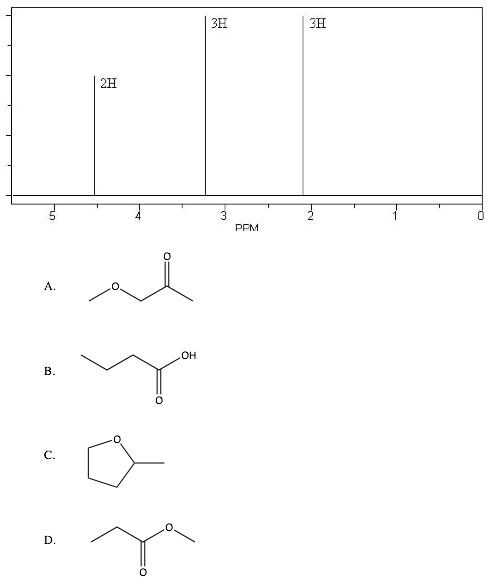Problem: An unknown compound W has the molecular formula C 4H8O2. Based on the following 1H NMR spectrum, what is the structure of compound W?

FREE Expert Solution
81% (395 ratings)
Problem Details

An unknown compound W has the molecular formula C 4H8O2. Based on the following 1H NMR spectrum, what is the structure of compound W?What scientific concept do you need to know in order to solve this problem?

Our tutors have indicated that to solve this problem you will need to apply the NMR Practice concept. You can view video lessons to learn NMR Practice Or if you need more NMR Practice practice, you can also practice NMR Practice practice problems .

What is the difficulty of this problem?

Our tutors rated the difficulty of An unknown compound W has the molecular formula C 4H8O2. Bas... as high difficulty.

How long does this problem take to solve?

Our expert Organic tutor, Chris took 5 minutes to solve this problem. You can follow their steps in the video explanation above.

What professor is this problem relevant for?

Based on our data, we think this problem is relevant for Professor Daoudi's class at UCF.